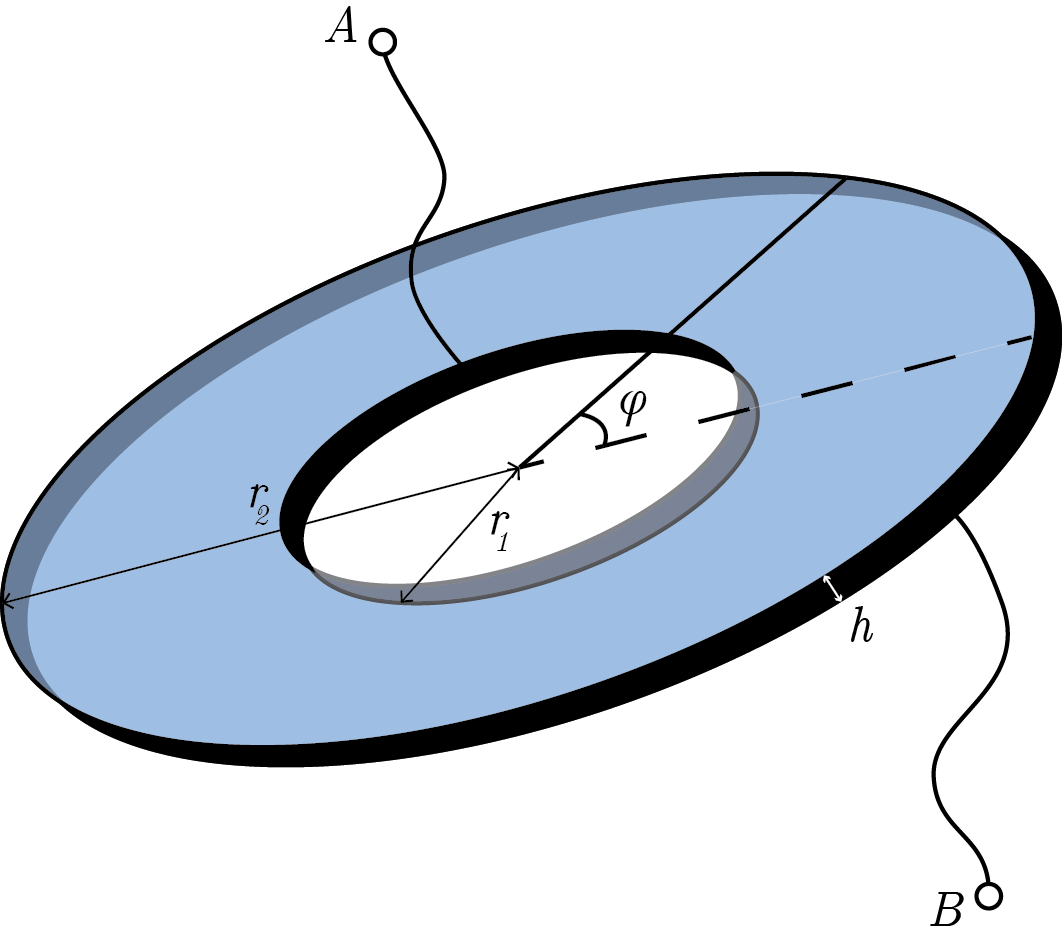# Can You Resist The Donut?You are given a disk of thickness $h$ with inner and outer radii $r_1$ and $r_2$, respectively. If the resistivity of the disk varies as $\rho = \rho_0 \left|\sec \varphi\right|$, where $\varphi$ is the polar angle, find the resistance between the points $A$ and $B$.

• Take $\dfrac {r_2}{r_1} = e^2 \approx 7.389$, $\rho_0 = \SI{10}{\ohm \meter}$, and $h= \SI{3}{\centi \meter}$.